Review your math skills in this quiz.

# Fractions (Dividing with Mixed Numbers)

This Math quiz is called 'Fractions (Dividing with Mixed Numbers)' and it has been written by teachers to help you if you are studying the subject at middle school. Playing educational quizzes is a fabulous way to learn if you are in the 6th, 7th or 8th grade - aged 11 to 14.

It costs only \$12.50 per month to play this quiz and over 3,500 others that help you with your school work. You can subscribe on the page at Join Us

Have you come to notice that when it comes to fractions, it seems that whether you are adding, subtracting, multiplying or dividing, in order to find the solution you have to use two or more of these methods? Perhaps this is one reason teachers really love to have their students work with fractions because they give the students lots of practice to keep the basic elements of math fresh in their minds. This quiz will keep you going on that road to practice.

Luckily, just as when you multiply with fractions there is no need to first find the “common denominator,” the same holds true with dividing fractions. And just as you did in multiplying fractions, in dividing you will only need to multiply the numerators together and then you multiply the denominators. However, before you can multiply the numerators and denominators, you must reverse or flip the second term in the problem. See below for a quick review of what you need to do.

Go straight to Quiz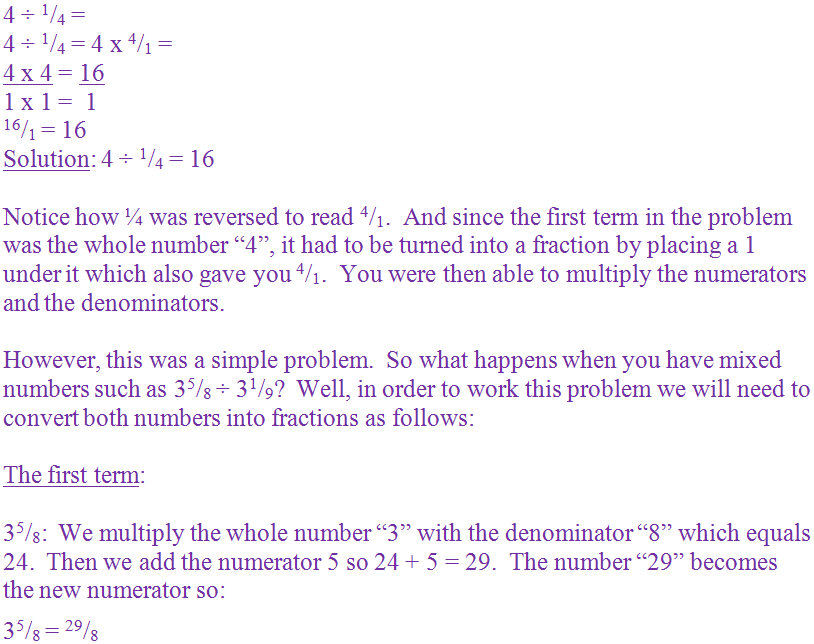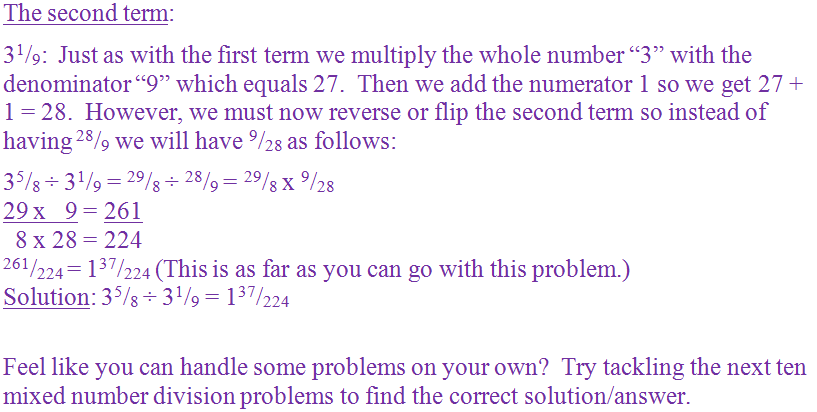1.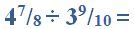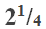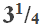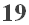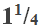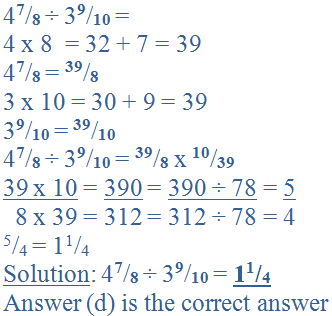2.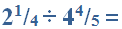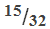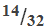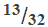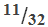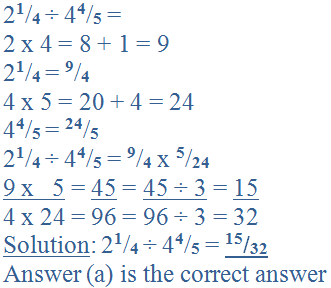3.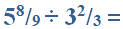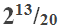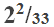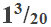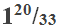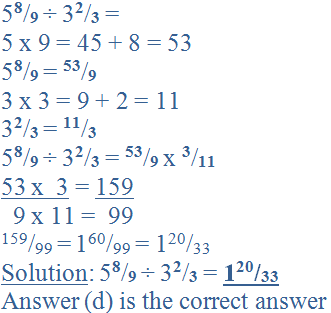4.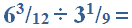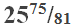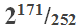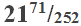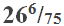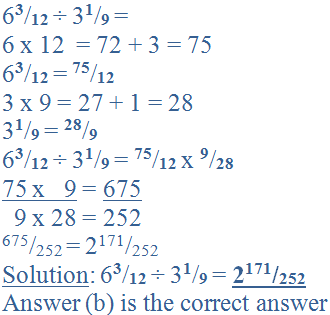5.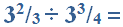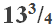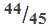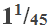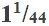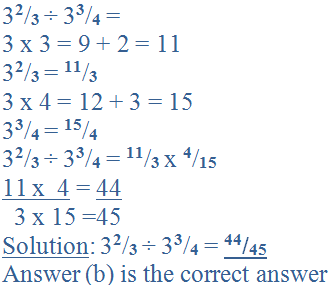6.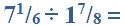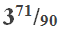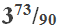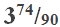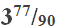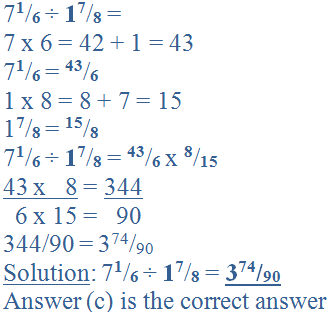7.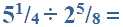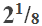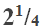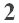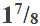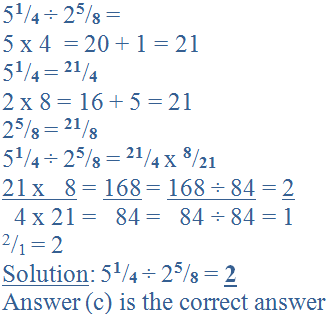8.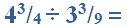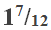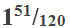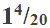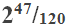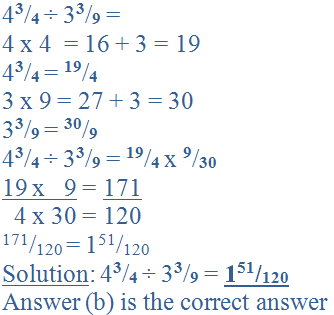9.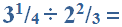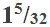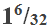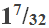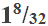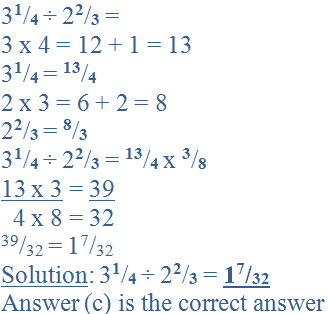10.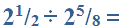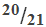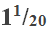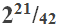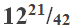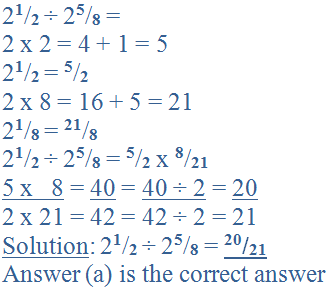Author:  Christine G. Broome